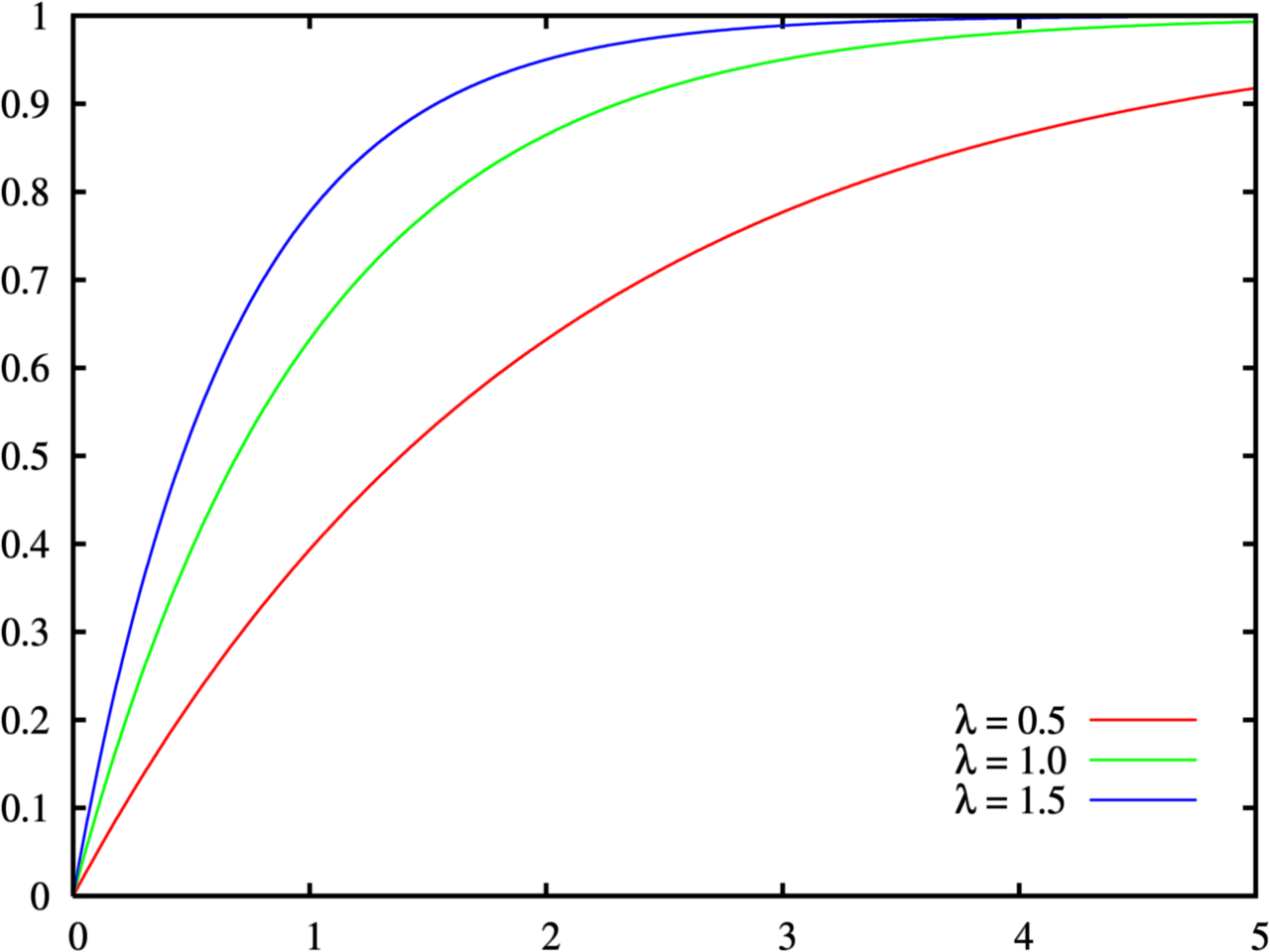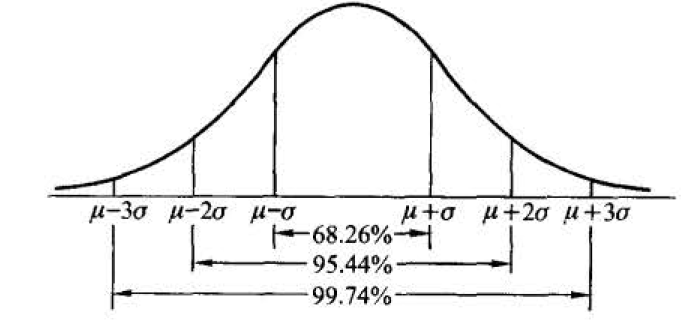# 第二章 随机变量及其分布

## 第二章 随机变量及其分布

- 样本空间 \rightarrow 函数的定义域
- 样本e \rightarrow 函数的自变量
- 实值单值函数X \rightarrow 函数关系
- 随机变量 \rightarrow 函数值

- 随机变量的取值随试验的结果而定，在试验之前不能预知它取什么值
- 且它的取值有一定的概率．这些性质显示了随机变量与普通函数有着本质的差异．

### 离散型随机变量及其分布律

P \lbrace X=x_{k} \rbrace = p_{k}, k=1,2, \cdots .

\begin{aligned}
& 1^{\circ} \quad p_{k} \geqslant 0, k=1,2, \cdots ; \\
& 2^{\circ} \sum_{k=1}^{\infty} p_{k}=1 . \\
\end{aligned}

2^{\circ} 是由于 \lbrace X=x_{1} \rbrace \bigcup \lbrace X=x_{2} \rbrace \cup \cdots 是必然事件, 且 \lbrace X=x_{j} \rbrace \cap \lbrace X=x_{k} \rbrace
= \varnothing, k \neq j
,故 1=P \left[ \bigcup_{k=1}^{\infty} \lbrace X=x_{k} \rbrace \right]=\sum_{k=1}^{\infty} P \lbrace X=x_{k} \rbrace \text {, 即 } \sum_{k=1}^{\infty} p_{k}=1.

#### (0 - 1)分布

P\lbrace X = k \rbrace = p^{k}(1-p)^{1-k}, k = 0,1 \quad (0<p<1),

#### 伯努利试验、二顶分布X \sim b(n,p)

\underbrace{p \cdot p \cdot \cdots \cdot p}_{k \uparrow} \cdot \underbrace{(1-p) \cdot(1-p) \cdot \cdots \cdot(1-p)}_{n-k \text { 个 }}=p^{k}(1-p)^{n-k} .

P \lbrace X=k \rbrace =\left(\begin{array}{l}
n \\
k \end{array}\right) p^{k} q^{n-k}, k=0,1,2, \cdots, n .

#### 泊松分布

P \lbrace X = k \rbrace = \frac{ \lambda^{ k } \mathrm{ e }^{- \lambda }} {k !}, k=0,1,2, \cdots,

n,p为参数的二项分布的概率值可以由参数为\lambda = np 的泊松分布的概率值近似．

\lim _{n \rightarrow \infty}\left(\begin{array}{l}
n \\\
k
\end{array}\right) p_{n}^{k}\left(1-p_{n}\right)^{n-k}=\frac{\lambda^{k} \mathrm{e}^{-\lambda}}{k !}

### 随机变量的分布函数

P \lbrace x_{1}<X \leqslant x_{2} \rbrace =P \lbrace X \leqslant x_{2} \rbrace -P \lbrace X \leqslant x_{1} \rbrace,

F(x)=P \lbrace X \leqslant x \rbrace,-\infty<x<\infty

F(x)是取值在区间[0,1]的不减函数。

### 连续型随机变量的概率密度及其分布

F(x)=\int_{-\infty}^{x} f(t) \mathrm{d} t,

1^{\circ} f(x) \geqslant 0;

2^{\circ} \int_{-\infty}^{\infty} f(x) \mathrm{d} x=1;

3^{\circ} 对于任意实数 x_{1}, x_{2}\left(x_{1} \leqslant x_{2}\right),

P \lbrace x_{1}<X \leqslant x_{2} \rbrace =F\left(x_{2}\right)-F\left(x_{1}\right)=\int_{-x_{1}}^{x_{2}} f(x) \mathrm{d} x,

4^{\circ}f(x) 在点 x 处连续, 则有 F^{\prime}(x)=f(x).

#### 均匀分布 X \sim U(a,b)

f(x)= \begin{cases}\frac{1}{b-a},&a<x<b, \ 0,&\text { 其他, }\end{cases}

X 在区间 (a, b) 上服从均匀分布. 记为 X \sim U(a, b).#### 指数分布

f(x)= \begin{cases}\frac{1}{\beta} \mathrm{e}^{-x / \beta},&x>0, \ 0,&\text { 其他, }\end{cases}

F(x ; \beta)=\lbrace \begin{array}{cl}
1-e^{- \frac{1}{\beta} x}&, x > 0 \\
0&, x<0
\end{array} .#### 正态分布/高斯分布X \sim N\left(\mu, \sigma^{2}\right)

f(x)=\frac{1}{\sqrt{2 \pi \sigma}} \mathrm{e}^{-\frac{(x-\mu)^{2}}{2 \sigma^{2}}},-\infty<x<\infty,

\begin{gathered}
\varphi(x)=\frac{1}{\sqrt{2 \pi}} \mathrm{e}^{-t^{2} / 2}, \\
\Phi(x)=\frac{1}{\sqrt{2 \pi}} \int_{-\infty}^{x} \mathrm{e}^{-t^{2} / 2} \mathrm{~d} t .
\end{gathered}

\Phi(-x) = 1-\Phi(x)